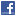## Relativity and Cosmology## Cosmos 2.0 - An Innovative Description of the Universe

Authors: Helmut Söllinger

The author's starting point is the axiom that the product of particle masses and the gravitational constant is constant and that the masses of the proton and the electron would increase while the gravitational constant would reduce over time. With this assumption, the author manages to explain the cosmic redshift without presupposing an expansion of the cosmos. He presents an underlying relation between the fundamental natural constants, the Hubble constant and the two particle masses, which would describe in quantitative terms not just the beginning, but also – and this is what is momentously new about this model – the end of the universe as we know it. Via a mathematical link, the temporal change in the gravitational constant and the particle masses is described in relation to the Hubble constant. In this context, attention must also be paid to the way in which the change of the electron mass affects the definition of the SI units for length and time. In addition, the author uses an analogy between magnetism and electricity in which the electron and the proton are considered as the two ends of an electrical dipole string. He develops a model for the elementary unit of the so-called dark matter through which the rotation characteristics of galaxies can be explained. On the basis of this assumed fundamental relation, the author quantifies the mass and length of the supposed elementary electrets, thus estimating the number of elementary electrets that should cross a typical galaxy. With his model, the author is the first to design a concrete and compact proposal for solving the greatest mysteries of cosmology.